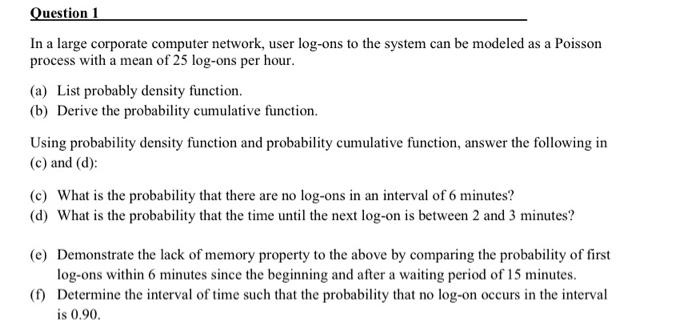# Question Solved1 AnswerQuestion 1 In a large corporate computer network, user log-ons to the system can be modeled as a Poisson process with a mean of 25 log-ons per hour. (a) List probably density function. (b) Derive the probability cumulative function. Using probability density function and probability cumulative function, answer the following in (c) and (d): (C) What is the probability that there are no log-ons in an interval of 6 minutes? (d) What is the probability that the time until the next log-on is between 2 and 3 minutes? (e) Demonstrate the lack of memory property to the above by comparing the probability of first log-ons within 6 minutes since the beginning and after a waiting period of 15 minutes. (1) Determine the interval of time such that the probability that no log-on occurs in the interval is 0.90.B3SM7O The Asker · Computer ScienceTranscribed Image Text: Question 1 In a large corporate computer network, user log-ons to the system can be modeled as a Poisson process with a mean of 25 log-ons per hour. (a) List probably density function. (b) Derive the probability cumulative function. Using probability density function and probability cumulative function, answer the following in (c) and (d): (C) What is the probability that there are no log-ons in an interval of 6 minutes? (d) What is the probability that the time until the next log-on is between 2 and 3 minutes? (e) Demonstrate the lack of memory property to the above by comparing the probability of first log-ons within 6 minutes since the beginning and after a waiting period of 15 minutes. (1) Determine the interval of time such that the probability that no log-on occurs in the interval is 0.90.
More
Transcribed Image Text: Question 1 In a large corporate computer network, user log-ons to the system can be modeled as a Poisson process with a mean of 25 log-ons per hour. (a) List probably density function. (b) Derive the probability cumulative function. Using probability density function and probability cumulative function, answer the following in (c) and (d): (C) What is the probability that there are no log-ons in an interval of 6 minutes? (d) What is the probability that the time until the next log-on is between 2 and 3 minutes? (e) Demonstrate the lack of memory property to the above by comparing the probability of first log-ons within 6 minutes since the beginning and after a waiting period of 15 minutes. (1) Determine the interval of time such that the probability that no log-on occurs in the interval is 0.90.